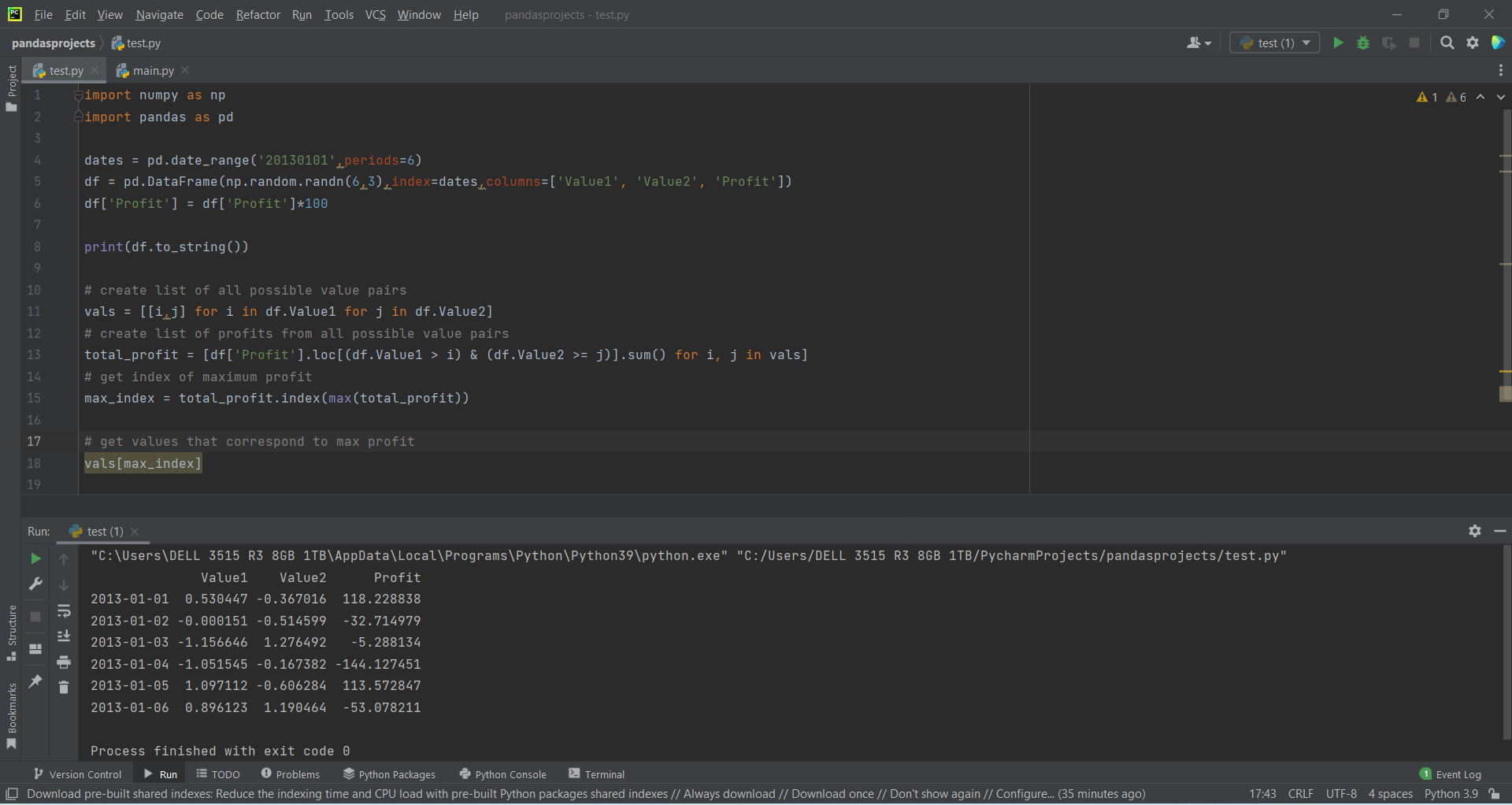# Profit and Linear Optimization using Pandasby Abdul Rawoof A RUpdated: Jul 31, 2023Solution Kit

Linear programming is a subset of optimization and method. It locates an optimal value for a linear objective function using a varying decision.

Python can also be used to optimize parameters in a model to best-fit data, increase the profitability of a potential engineering design, or meet other objectives that can be described mathematically with the variables and equations in Python. The optimization process's essential goal is to find variables' values that minimize or maximize the objective function while satisfying the constraints. This result is called an optimal solution or optimal result. The optimizations generally have three components: objective functions, decision variables, and constraints. There are two different types of optimization or solving methods in Python,

• Linear optimization: It is a method that includes techniques known as tabu search and scatter search.
• Nonlinear optimization: It is a method that includes genetic algorithms.

Types of optimization models:

• Linear Programming(LP).
• Nonlinear Programming(NLP).
• Constraint Programming(CP).
• Mixed integer Programming(MIP).

Here is an example of how to implement profit and linear optimization in Pandas:Fig : Preview of the output that you will get on running this code from your IDE.

### Code

In this solution we're using Pandas and numpy libraries.

### Instructions

Follow the steps carefully to get the output easily.

2. Copy the snippet using the 'copy' and paste it in your IDE.
3. Add required dependencies and import them in Python file.
4. Run the file to generate the output.

I hope you found this useful. I have added the link to dependent libraries, version information in the following sections.

I found this code snippet by searching for 'profit and linear optimization using pandas' in kandi. You can try any such use case!

### Environment Tested

I tested this solution in the following versions. Be mindful of changes when working with other versions.

1. The solution is created in PyCharm 2021.3.
2. The solution is tested on Python 3.9.7.
3. Pandas version-v1.5.2.
4. numpy version-v1.24.0.

Using this solution, we are able to implement profit and linear optimization using pandas with simple steps. This process also facilities an easy way to use, hassle-free method to create a hands-on working version of code which would help us to implement profit and linear optimization using pandas.

### Dependent Libraries

pandasby pandas-dev

Python38689Version:v2.0.2Flexible and powerful data analysis / manipulation library for Python, providing labeled data structures similar to R data.frame objects, statistical functions, and much more

Support
Quality
Security
Reuse

pandasby pandas-dev

Python38689Version:v2.0.2License: Permissive (BSD-3-Clause)

Flexible and powerful data analysis / manipulation library for Python, providing labeled data structures similar to R data.frame objects, statistical functions, and much more
Support
Quality
Security
Reuse

numpyby numpy

Python23755Version:v1.25.0rc1The fundamental package for scientific computing with Python.

Support
Quality
Security
Reuse

numpyby numpy

Python23755Version:v1.25.0rc1License: Permissive (BSD-3-Clause)

The fundamental package for scientific computing with Python.
Support
Quality
Security